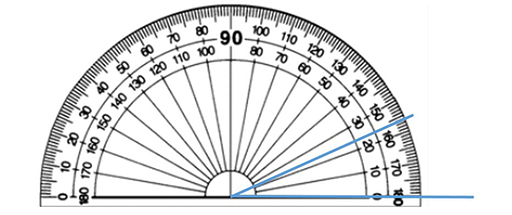Education & Development

### Become an OU studentTeaching mathematics

Start this free course now. Just create an account and sign in. Enrol and complete the course for a free statement of participation or digital badge if available.

# 3.1 Using a protractor to measure angles

The half-circle protractor is commonly used to measure angles (Figure 9). The inner number scale rises from 0 to 180, from right to left, and the outer scale goes from 0 to 180, left to right. Learners commonly make mistakes because they are not using the correct scale when measuring the angle between two lines. The point to remember when measuring angles is that you need to start at zero on one of the lines and read round on that scale to see the number on the second line.Figure 9 Using a protractor to measure an angle

In order to read the number from the protractor, it may help to use a ruler to make the lines longer so that they meet the scales on the protractor. Make sure that one of the lines in the angle sits on the zero line. Then, using the scale which starts at zero (the inner scale in this case), read off where the second line meets the scale (24⁰ for this particular angle). If a learner gets 156⁰ for the angle, it is obvious that they have made a mistake. Why is this obvious?

The angle is smaller than a right angle (acute), so the number of degrees must be less than 90.

Degrees in a circle

As you can see from the half-circle protractor displaying 180⁰, it is commonly accepted that there are 360⁰in a circle. No one is entirely sure where this originated, possibly with the Babylonians [2000-1600 BC]. It is certainly worth having at least one plausible explanation ready when teaching this in order to answer the question ‘Why?’